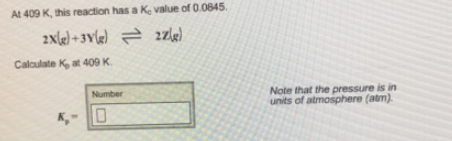# Problem: At 409 K, this reaction has a Kc value of 0.0845. 2X(g) + 3Y(g) ⇌ 2Z(g)Calculate Kp at 409 K.[Note that the pressure is in units of atmosphere (atm).]

###### FREE Expert Solution
100% (130 ratings)###### Problem Details

At 409 K, this reaction has a Kc value of 0.0845.

2X(g) + 3Y(g) ⇌ 2Z(g)

Calculate Kp at 409 K.

[Note that the pressure is in units of atmosphere (atm).]